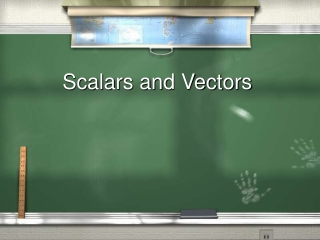DownloadDownload PresentationScalars and Vectors

# Scalars and Vectors

Download Presentation## Scalars and Vectors

- - - - - - - - - - - - - - - - - - - - - - - - - - - E N D - - - - - - - - - - - - - - - - - - - - - - - - - - -
##### Presentation Transcript

1. Scalars and Vectors

2. Scalars are quantities which are fully described by a size alone. Eg. Temperature, Distance, Time, Speed, Mass, Length

3. Vectors are quantities which are fully described by both a size and a direction. Eg. Velocity, Acceleration, Force, Thrust, Drag To properly describe a vector, you must include two items: Size or quantity and Direction. For example, 25m/s north.

4. Direction can also be given using a + and -. For example, +ve can be defined as west and –ve as east.

5. Graphically Representing Vectors Vectors are drawn using arrows. Arrows have size (length) The greater the size of the vector, the longer the arrow. Arrows have and show direction

6. If this vector below represents a velocity of 13 m/s, east, select the vector that represents 6.5 m/s, west. 13 m/s, E 6.5 m/s, W

7. Motion Concepts Motion means a continuous change in the position of a body relative to a reference point. Average Speedis defined as the total distance an object moved divided by the total time needed to make the move. Average Speed is a scalar quantity.

8. vs represents the average speed d is the distance Dt is the interval of time needed for the move

9. (the Greek lower case letter “delta”) is very important. It means a "change in". Dt is the difference between the time at the end of the motion tf (final time) and the time at the beginning of the motion ti (initial time).